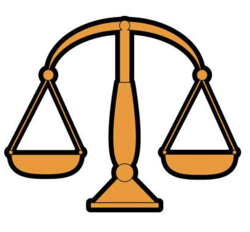#Minimum set of weight puzzle

This puzzle is a mix of weight and math puzzle.

## Puzzle

What is the minimum number of weights needed for a scale that will be able to weigh objects from the weight of one pound to 100 pounds?

## Solution

1. If only one side pan of the balance-scale is used,
Weights are : 2^n <100 i.e. {1,2,4,8,16,32,64} = 7

2. If both side pan of balance-scale is used,
Weights are : 3^ n <100 i.e. {1,3,9,27,81} = 5

##### Mathematical Explanation

For each weight, there are three things you can do:-
put it on the left pan, the right pan, or not on the balance at all.

So, if you have n weights, there are 3n things you can do with them.

One of those things is not putting any weights on the scale, which is good if you want to weigh a 0-pound object, so really there are only (3n – 1) arrangements.

Then, for each arrangement there’s also its mirror image (where all the weights are switched to the opposite pan of the scale), so there are at most (3n – 1)/2 arrangements of n weights.

That’s enough to prove that 4 weights can weigh at most 40 different things … 40 is really the upper limit for 4 weights.

With a fifth weight, you should be able to get up to (35 – 1)/2 =121 pounds.